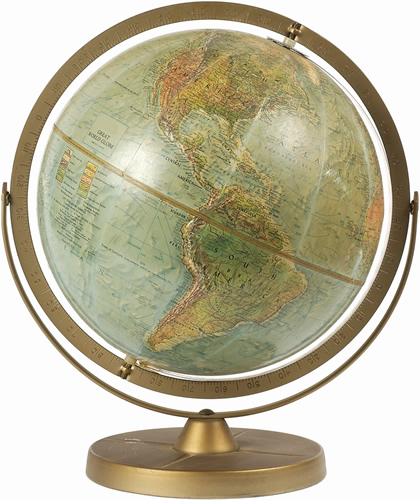• Math, 3rd QUARTER

Unit 9: Solve word problems /Money

In this unit of study students will identify coins and their values. Students will use the dollar symbol and the cents symbol appropriately.  Students will show multiple combinations of coins or dollars for a given value and make change. Students will solve word problems using either dollars or cents and relate to real-world applications.

In this unit students will utilize various models and strategies through multiple experiences to add and subtract 1, 2, and 3 digit numbers up to 1000.  Students will explore concrete and representational ways to compose and decompose numbers and solve multi-digit addition and subtraction problems.

In this unit students will expand their understanding for multiple interpretations of addition and subtraction solutions.  Students will explain and justify strategies used during computation and problem solving based on place value and properties of operations through multiple experiences.

In this unit students add and subtract to 20, applying an understanding of the properties of operations and the inverse relationship between addition and subtraction. They focus on using different strategies to fluently add and subtract numbers within 20 and work towards learning these problems from memory by the end of 2nd grade. Students will also use addition to find the total number of objects arranged in rectangular arrays with up to 5 rows and up to 5 columns; write an equation to express the total as a sum of equal addends.

Unit 13: Two three digit numbers

In this unit students will understand that place value is the key to comparing two and three digit numbers.  They will learn comparison symbols and will use mathematical symbols to compare and record two and three digit numbers through multiple experiences.

End of 3rd quarter assessment

Language Arts

RL.2.1 Ask and answer such questions as who, what, where, when, why, and how to demonstrate understanding of key details in a text.

RI.2.1 Ask and answer such questions as who, what, where, when, why, and how to demonstrate understanding of key details in a text.

RI 2.2 Identify the main topic of a multi-paragraph text as well as the focus of specific paragraphs within the text.

RI.2.3 Describe the connection between a series of historical events, scientific ideas or concepts, or steps in technical procedures in a text.

RI.2.4 Determine the meaning of words and phrases in a text relevant to a grade 2 topic or subject area.

RI.2.5 Know and use various text features (e.g., captions, bold print, subheadings, glossaries, indexes, electronic menus, icons) to locate key facts or information in a text efficiently.

RI.2.6  Identify the main purpose of a text, including what the author wants to answer, explain, or describe.

RI.2.7  Explain how specific images (e.g., a diagram showing how a machine works) contribute to and clarify a text.

RI.2.8  Describe how reasons support specific points the author makes in a text.

Social Studies/Science

Q3 Weather/Matter

Let's do all this in two languages while learning with a focus on Asia. Speas is  a global school!!!!